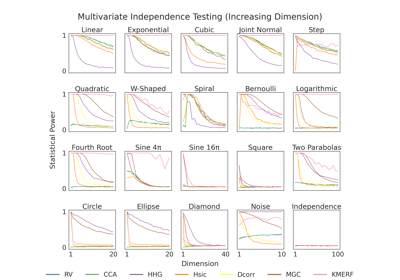# power¶

hyppo.tools.power(test, sim_type, sim=None, n=100, alpha=0.05, reps=1000, auto=False, **kwargs)

Computes empircal power for hypothesis tests

Parameters
• test (str or list) -- The name of the independence test (from the hyppo.independence module) that is to be tested. If MaxMargin, accepts list with first entry "MaxMargin" and second entry the name of another independence test. For hyppo.ksample.KSample put the name of the independence test. For other tests in hyppo.ksample just use the name of the class.

• sim_type ("indep", "ksamp", "gauss") -- Type of power method to calculate. Depends on the type of sim.

• sim (str, default: None) -- The name of the independence simulation (from the hyppo.tools module). that is to be used. Set to None if using Gaussian simulation curve.

• n (int, default: 100) -- The number of samples desired by the simulation (>= 5).

• alpha (float, default: 0.05) -- The alpha level of the test.

• reps (int, default: 1000) -- The number of replications used to estimate the null distribution when using the permutation test used to calculate the p-value.

• auto (bool, default: False) -- Automatically uses fast approximation when n and size of array is greater than 20 or test has a non-permutation based p-value. If True, and sample size is greater than 20, then hyppo.tools.chi2_approx will be run. reps is irrelevant in this case. See documentation for test if this parameter applies.

• **kwargs -- Additional keyword arguements for sim.

Returns

empirical_power (ndarray) -- Estimated empirical power for the test.

## Examples using hyppo.tools.power¶